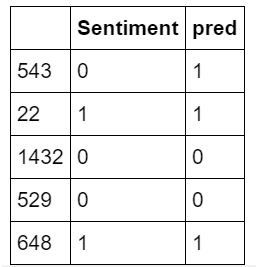top of page
Search

# Sentiment AnalysisSentiment analysis is the process of extraction of emotions behind textual data. A sentiment analyzer is a widely adopted tool in most business sectors. Some of the common applications of sentiment analyzers are listed below:

2. Enhancing the Customer Experience through Sentiment Analysis in Business

3. Sentiment Analysis in Business for Brand Brisking.

Sentiment Analysis is the most common text classification tool that analyses an incoming message and tells whether the underlying sentiment is positive, negative, or neutral.

### Environment Setup:

The project is set up in Anaconda Environment on the jupyter notebook.

### Dependencies/Libraries Required:

• pandas

• sklearn

• pickle

• nltk

• matplotlib

• word cloud

• seaborn

1. Dataset Exploration: The first step is the Dataset Exploration step which includes the process of loading a dataset and checking out its fields with a bit of visualization.

2. Preparation and Feature Engineering: This step includes the removal of stopword and other basic preprocessing. In Feature Engineering raw dataset is transformed into vector formations that can be used by the machine learning model.

3. Model Training: The final step is the Model Building step in which a machine learning model is trained on a labeled dataset.

4. Evaluation of Text Classifier: The Classifier could be evaluated using different evaluation measures such as confusion matrix, F1-Score, Accuracy score, etc.

### Importing The Libraries:

```%matplotlib inline
from sklearn import metrics
import seaborn as sn
import pandas as pd
from sklearn.feature_extraction.text import CountVectorizer
import pickle
import nltk
from sklearn.model_selection import train_test_split
from sklearn.metrics import classification_report, f1_score,accuracy_score
from wordcloud import WordCloud
import matplotlib.pyplot as plt
from sklearn import model_selection, preprocessing,svm```

In this step, we imported all the required libraries like seaborn, pandas(for preprocessing). nltk(For textual) etc.

### Data Exploration:

Once the environment is set up and dependencies are installed it is time to get started and explore our data set. For this particular article, I have used a dataset consisting of more than 1000000 textual sentences along with their respective targets. The targets, in this case, are the sentiments which are positive and negative. So this becomes a binary classification problem.

```data = pd.read_csv(dataset,engine='python')

In this above code file, we imported our dataset with moreover 1M of data.

Here is how the dataset looks likeIn this dataset

ItemID: Represents the Serial No.

Sentiment: Represents whether the text sentiment is positive or negative.

0 shows negative sentiment whereas 1 shows positive sentiment.

SentimentText: Represents the texts(For which we need to build our model to check further text sentiments).

### Wordcloud:

In this Step, the word cloud has built on column SentimentText.

```all_words = ' '.join([text for text in data['SentimentText']])
wordcloud = WordCloud(width=800, height=500, random_state=21, max_font_size=110).generate(all_words)
plt.figure(figsize=(10, 7))
plt.imshow(wordcloud, interpolation="bilinear")
plt.axis('off')
plt.show()```In this section, the word cloud has made on column SentimentText.

In the first step, all words are joined. Then a word cloud with height 800 and width 500, with font size 110 has been plotted. (With figure size of width 10 and height 7) the word cloud interpolation is bilinear.

### Data Preparation & Feature Engineering:

This step, we need to remove stopwords, Punctuations, exclamation marks, convert uppercase to lowercase, etc. Punctuation, numbers, and special characters do not help much. It is better to remove them from the text.

Let's Look at the rows.

```data_new = data.iloc[:3000]we can see some stopwords, ... , we need to remove those for building a good and better model.

```data.replace(r'\b\w{1,4}\b','', regex =True, inplace = True)
all_words = ' '.join([text for text in data_new['SentimentText']])
wordcloud = WordCloud(width=800, height=500, random_state=21, max_font_size=110).generate(all_words)
plt.figure(figsize=(10, 7))
plt.imshow(wordcloud, interpolation="bilinear")
plt.axis('off')
plt.show()```

So here we replace those entities from the data, and then join with all data. and we can see that this word cloud is more accurate than the previous one.### Train test Split:

The next part will be to convert it to a vectorize format and split the dataset into training and testing part.

```vectorizer = CountVectorizer()
vectorizer.fit(data_new['SentimentText'])
vec = vectorizer.transform(data_new['SentimentText'])
data['encoded_text'] = vec
Train_X, Test_X, Train_Y, Test_Y = model_selection.train_test_split(vec,data_new['Sentiment'],test_size=0.1)Let's check the shape of the training and testing data.

`Train_X.shape,Test_X.shape`

((2700, 6681), (300, 6681))

### Model Training:

This involves the selection of algorithms and training models based on that algorithm. There are multiple algorithms that could perform this kind of stuff e.g Naive Bayes, SVM, Neural nets, and so on.

```SVM = svm.SVC(C=1.0, kernel='linear', degree=3, gamma='auto')
SVM.fit(Train_X , Train_Y)
predictions_SVM = SVM.predict(Test_X)```

here we have imported the Support vector machine model into it to train our model.

### Model Evaluation:

The accuracy of 76.66 with an F1-score of 0.76 is achieved by SVM, which is not that bad we can tune this model and choose different features like POS, word embeddings, etc in place of cout vector formations in order to increase the accuracy and other evaluation measures of our model.

`print("SVM Accuracy Score -> ",accuracy_score(predictions_SVM,Test_Y)*100)print(classification_report(Test_Y,predictions_SVM))print(f1_score(Test_Y,predictions_SVM,average='weighted'))`

SVM Accuracy Score -> 76.33333333333333 precision recall f1-score support 0 0.84 0.85 0.84 225 1 0.53 0.51 0.52 75 accuracy 0.76 300 macro avg 0.68 0.68 0.68 300 weighted avg 0.76 0.76 0.76 300 0.7617020318061

### Confusion Matrix:

```cm=metrics.confusion_matrix(Test_Y,predictions_SVM)
plt.matshow(cm)``````plt.figure(figsize = (10,7))
ax= plt.subplot()
ax.set_title('Confusion Matrix');
sn.heatmap(cm, annot=True,ax = ax)```

Here the heatmap of the confusion matrix is plotted.Let's see the confusion matrix

`cm`

array([[191, 34], [ 37, 38]])

So here we got 72 incorrect predictions and 228 incorrect predictions.

Compare the True vs Predicted

```df = pd.DataFrame(Test_Y)
df['pred'] = predictions_SVM
sent = df['Sentiment']
pred = df['pred']Let's analyze the positive and negative sentiments.

```plt.title('Sentiment distribution')
cat = ['positive', 'negative']
freq = [len(negative),len(positive)]
plt.ylabel('frequency')
plt.bar(cat,freq,color= ['blue','green'])
plt.show()```So in this manner, we can build the sentiment analysis.

For More Reference Check this GitHub Link: https://github.com/kapuskaFaizan/NLP-jupyter_notebook/blob/master/Sentiment_analysis.ipynb

Thank You!